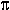DOC HOME SITE MAP MAN PAGES GNU INFO SEARCH PRINT BOOK
Programming with awk

# Arithmetic

Actions can use conventional arithmetic expressions to compute numeric values. As a simple example, suppose you want to print the population density for each country in the file countries. Since the second field is the area in thousands of square miles and the third field is the population in millions, the expression 1000\$3 / \$2 gives the population density in people per square mile. The program

```   { printf "%10s %6.1f\n", \$1, 1000 * \$3 / \$2 }
```
when applied to the file countries, prints the name of each country and its population density:
```         USSR   30.3
China  234.6
USA   60.6
Brazil   35.3
Australia    4.7
India  502.0
Argentina   24.3
Sudan   19.6
Algeria   19.6
```

Arithmetic is done internally in floating point. The arithmetic operators are +, -,, /, % (remainder) and ^ (exponentiation;is a synonym). Arithmetic expressions can be created by applying these operators to constants, variables, field names, array elements, functions, and other expressions, all of which are discussed later. Note that awk recognizes and produces scientific (exponential) notation: 1e6, 1E6, 10e5, and 1000000 are numerically equal.

awk has assignment statements like those found in the C programming language. The simplest form is the assignment statement

```   v = e
```
where v is a variable or field name, and e is an expression. For example, to
compute the number of Asian countries and their total population, you could write
```   \$4 == "Asia"  { pop = pop + \$3; n = n + 1 }
END           { print "population of", n,
"Asian countries in millions is", pop }
```
Applied to countries, this program produces
```   population of 3 Asian countries in millions is 1765
```
The action associated with the pattern \$4 == "Asia" contains two assignment statements, one to accumulate population and the other to count countries. The variables are not explicitly initialized, yet everything works properly because awk initializes each variable with the string value "" and the numeric value 0.

The assignments in the previous program can be written more concisely using the operators += and ++:

\$4 == "Asia" { pop += \$3; ++n }

The operator += is borrowed from the C programming language; therefore,

```   pop += \$3
```
has the same effect as
```   pop = pop + \$3
```
but the += operator is shorter and runs faster. The same is true of the ++ operator, which adds one to a variable.

The abbreviated assignment operators are +=, -=,=, /=, %=, and ^=. Their meanings are similar:

v op= e

has the same effect as

v = v op e.

The increment operators are ++ and --. As in C, they may be used as prefix (++x) or postfix (x++) operators. If x is 1, then i=++x increments x, then sets i to 2, while i=x++ sets i to 1, then increments x. An analogous interpretation applies to prefix and postfix --.

Assignment and increment and decrement operators may all be used in arithmetic expressions.

We use default initialization to advantage in the following program, which finds the country with the largest population:

```   maxpop < \$3  { maxpop = \$3; country = \$1 }
END          { print country, maxpop }
```
Note, however, that this program would not be correct if all values of \$3 were negative.

awk provides the built-in arithmetic functions listed in ``awk built-in arithmetic functions''.

awk built-in arithmetic functions

Function Value returned
atan2(y,x) arctangent of y/x in the range -tocos(x) cosine of x, with x in radians
exp(x) exponential function of x
int(x) integer part of x truncated towards 0
log(x) natural logarithm of x
rand() random number between 0 and 1
sin(x) sine of x, with x in radians
sqrt(x) square root of x
srand(x) x is new seed for rand

x and y are arbitrary expressions. The function rand returns a pseudo-random floating point number in the range (0,1), and srand(x) can be used to set the seed of the generator. If srand has no argument, the seed is derived from the time of day.

Next topic: Strings and string functions
Previous topic: awk built-in variables